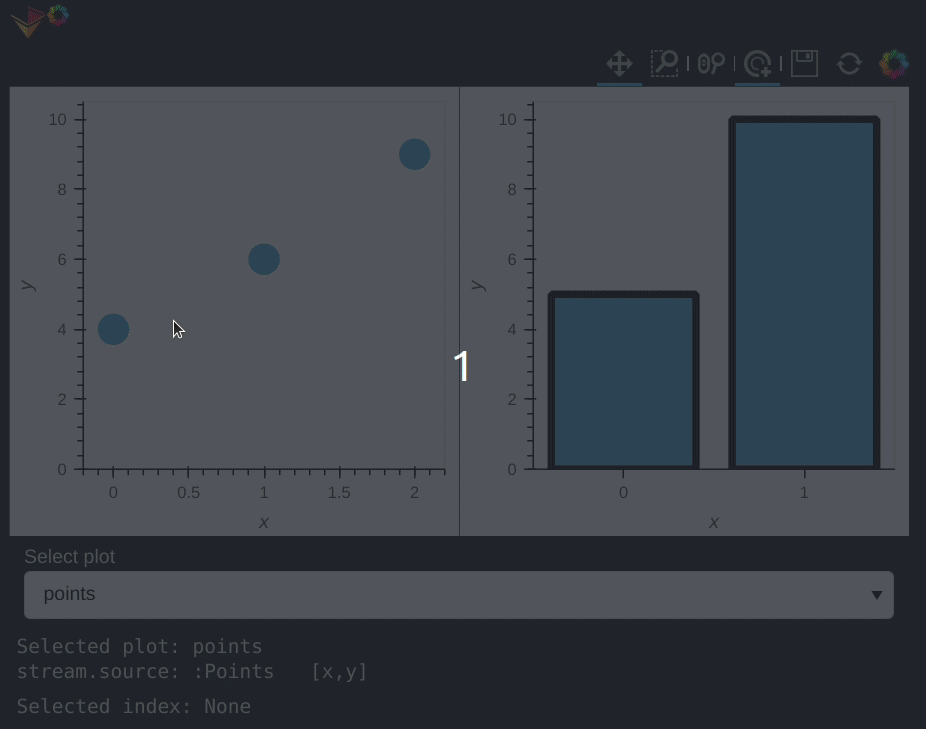# How can I update the source of a Holoviews stream with respect to Panel dependencies?

I have a Panel dependency on a Holoviews stream. I need to update the stream’s source to change between different Holoviews plots. When I update the stream source this is reflected in `stream.source`, however the Panel dependency acts just like the stream source is not updated.

For example this minimal example:

``````import numpy as np
import holoviews as hv
import panel as pn
import param

hv.extension('bokeh')
pn.extension()

points = hv.Points(
(np.array([0, 1]), np.array([4, 9]))
).opts(
size=15,
)

bars = hv.Bars(
(np.array([0, 1]), np.array([5, 10]))
).opts(
line_width=5,
)

stream = hv.streams.Selection1D(source=points, index=None)

select_plot = pn.widgets.Select(
name='Select plot',
options=["points", "bars"],

)

@pn.depends(stream.param.index)
def selection(index):
"""Panel dependency on the stream"""
return pn.pane.Str(f'Selected index: {index}', width=500, height=15)

@pn.depends(select_plot.param.value)
def update_stream_source(val):
"""Update Stream source plot"""
if val == "points":
stream.source = points
elif val == "bars":
stream.source = bars
else:
stream.source = None
return pn.pane.Str(f'Selected plot: {val}\nstream.source: {stream.source}', width=500, height=30)

pn.Column(
(points.opts(tools=["tap"]) + bars.opts(tools=["tap"])),
select_plot,
update_stream_source,
selection,

)
``````

results in:Is it possible to update the stream source and also making sure that the Panel dependencies are respected with respect to the update?

Versions used:

• Holoviews version: 1.15.0
• Panel version: 0.13.1
• Param version: 1.12.2